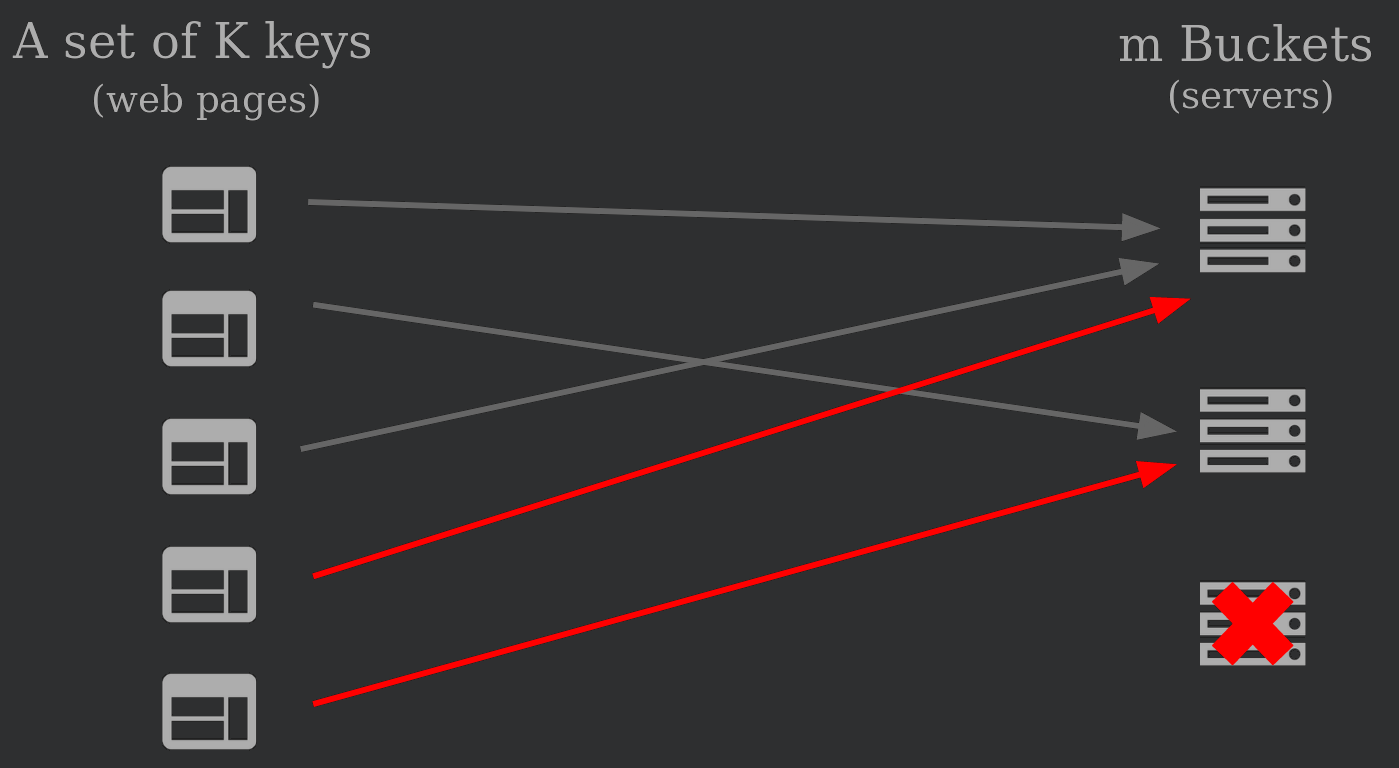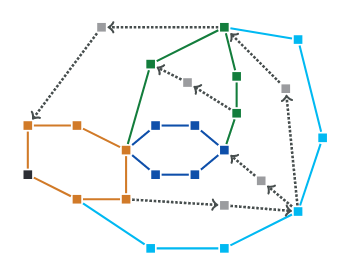# Tag: Computer Science

• ## Presentation on consistent hashing (ARPE)Here are the sides of a presentation I did on consistent hashing that I did to prepare for my year of reasearch in Pisa. In consistent hashing you want to be able to assign dinamically a set of keys K to a set of buckets,in which they will be stored, and the number of bucket can either increase or decrease at any moment and the keys should be remapped efficiently (less tha K/m remapping in averrage, m being the number of buckets).

• ## A recap of 3 articles of FOCS 2018

I had the chance to volunteer at the FOCS 2018 conference and here are 3 articles that I specially enjoyed and I give a short summary of them here.

### Bloom Filters, Adaptivity, and the Dictionary Problem

The dictionary problem considers a big dictionary $S$ on which you want to be able to know if a word $x$ is in $S$. The entire dictionary, because of its size, is usually saved remotely and you want to avoid querying it as much as possible. By using an approximate membership query data structure (AMQ) you can answer pre-queries that always answer PRESENT if $x \in S$ but also has false positive.

This paper proposes to use Bloom filters to create an adaptive filter (the filters adapts to an $\epsilon$ probability of having a false-positive), this method gives very good guarantees on false positives without significant space cost. Their contributions are 2 main results:

• When no feedback can be obtain on positive queries, adaptivity is impossible.
• It is possible to maintain an adaptive AMQ for the same cost as a non adaptative AMQ.

You can find the final paper here.

### Limits on All Known (and Some Unknown) Approaches to Matrix Multiplication

Determining matrix multiplication’s complexity is a fundamental problem in theoretical computer science. The naive algorithm is in $\mathcal{O}(n^3)$ and the famous Strassen algorithm runs in $\mathcal{O}(n^{2.8074})$. The problem is to find the minimum $\omega$ such that matrix multiplication (MM) can be solved in time $\mathcal{O}(n^{\omega})$, more specifically, to know whether $\omega=2$… The best bound on $\omega$ so far is close to $2.3$.

This paper first unites the two main approaches : Laser method of Strassen, and the Group theoretic approach of Cohn and Umans. This new technique, based on zeroing out some variable of the tensor, is called the \textbf{Solar method}. Then, they introduce two more general methods, one based on monomial degeneration, the Galactic method, and the other where any degeneration is allowed, the Universal method.

After introducing those 3 methods, the paper shows that to find a method where $\omega$ could potentially be equal to 2, you have to search for a Universal method because the Solar and Galactic method aren’t powerful enough to provide such a small lower bound.

You can find the final paper here.

### Beating the integrality ratio for s-t-tours in graphs

The Travelling Salesman Problem (TSP) is well known and the s-t path variant of the TSP (shortest tour from $s$ to $t$ that goes through all vertices) has one advantage, it has an well-known 1.5 approximation-ratio. In this paper by detailing special cases of ears configuration and having a details analysis of those cases they beat the approximation ratio 1.5, and get a 1.497 approximation ratio.An example of a well-oriented ear-decomposition.

You can find the final paper here.